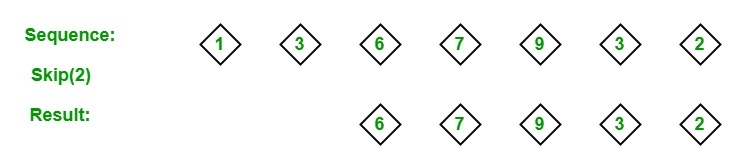Open in App
Not now

# LINQ | Partition Operator | Skip

• Last Updated : 28 May, 2019

In LINQ, partition operators are used for separating the given sequence into two portions without sorting the elements and return one of the portions. The Standard Query Operators supports 4 different types of partition operators:

1. Skip
2. SkipWhile
3. Take
4. TakeWhile

#### Skip Operator

The Skip operator is used to skips the elements up to the specified position in the given sequence or collection. Or in other words, we can say that it skips the specified number of elements and return the remaining elements present in the given collection or sequence.• It does not support query syntax in C# and VB.Net languages. But you can use the Skip method to query variable or you can wrap your query in brackets and then the call Skip method.
• It support method syntax in both C# and VB.Net languages.
• It present in both the Queryable and Enumerable class.
• It is implemented by using deferred execution.
• It will throw an ArgumentNullException if the source is null.

Example 1:

 `// C# program to illustrate the  ` `// concept of Skip operator ` `using` `System; ` `using` `System.Linq; ` ` `  `class` `GFG { ` ` `  `    ``static` `public` `void` `Main() ` `    ``{ ` ` `  `        ``// Data source ` `        ``char``[] sequence1 = {``'c'``, ``'y'``, ``'p'``, ` `                           ``'q'``, ``'l'``, ``'a'``}; ` ` `  `        ``int``[] sequence2 = {202, 2002, 20002, ` `                           ``200002, 2000002}; ` ` `  `        ``// Display the sequences ` `        ``Console.WriteLine(``"Original Sequence 1 is: "``); ` ` `  `        ``foreach``(``var` `s1 ``in` `sequence1) ` `        ``{ ` `            ``Console.WriteLine(s1); ` `        ``} ` ` `  `        ``// Query which skips starting 4 ` `        ``// elements of the sequence1 ` `        ``// Using Skip method ` `        ``var` `result1 = sequence1.Skip(4); ` `        ``Console.WriteLine(``"New Sequence: "``); ` ` `  `        ``// Display new sequence ` `        ``foreach``(``var` `val ``in` `result1) ` `        ``{ ` `            ``Console.WriteLine(val); ` `        ``} ` ` `  `        ``Console.WriteLine(``"Original Sequence 2 is: "``); ` ` `  `        ``foreach``(``var` `s2 ``in` `sequence2) ` `        ``{ ` `            ``Console.WriteLine(s2); ` `        ``} ` ` `  `        ``// Query which skip starting 2  ` `        ``// elements of the sequence2 ` `        ``// Using Skip method ` `        ``var` `result2 = sequence2.Skip(2); ` ` `  `        ``Console.WriteLine(``"New Sequence: "``); ` ` `  `        ``// Display new sequence ` `        ``foreach``(``var` `val ``in` `result2) ` `        ``{ ` `            ``Console.WriteLine(val); ` `        ``} ` `    ``} ` `} `

Output:

```Original Sequence 1 is:
c
y
p
q
l
a
New Sequence:
l
a
Original Sequence 2 is:
202
2002
20002
200002
2000002
New Sequence:
20002
200002
2000002
```

Example 2:

 `// C# program to find the Id's of ` `// the employees starting from 211 ` `using` `System; ` `using` `System.Linq; ` `using` `System.Collections.Generic; ` ` `  `// Employee details ` `public` `class` `Employee { ` ` `  `    ``public` `int` `emp_id ` `    ``{ ` `        ``get``; ` `        ``set``; ` `    ``} ` ` `  `    ``public` `string` `emp_name ` `    ``{ ` `        ``get``; ` `        ``set``; ` `    ``} ` ` `  `    ``public` `string` `emp_gender ` `    ``{ ` `        ``get``; ` `        ``set``; ` `    ``} ` ` `  `    ``public` `string` `emp_hire_date ` `    ``{ ` `        ``get``; ` `        ``set``; ` `    ``} ` ` `  `    ``public` `int` `emp_salary ` `    ``{ ` `        ``get``; ` `        ``set``; ` `    ``} ` `} ` ` `  `public` `class` `GFG { ` ` `  `    ``// Main method ` `    ``static` `public` `void` `Main() ` `    ``{ ` ` `  `        ``List emp = ``new` `List() { ` ` `  `            ``new` `Employee() {emp_id = 209, emp_name = ``"Anjita"``, emp_gender = ``"Female"``, ` `                                    ``emp_hire_date = ``"12/3/2017"``, emp_salary = 20000}, ` ` `  `            ``new` `Employee() {emp_id = 210, emp_name = ``"Soniya"``, emp_gender = ``"Female"``, ` `                                    ``emp_hire_date = ``"22/4/2018"``, emp_salary = 30000}, ` ` `  `            ``new` `Employee() {emp_id = 211, emp_name = ``"Rohit"``, emp_gender = ``"Male"``, ` `                                  ``emp_hire_date = ``"3/5/2016"``, emp_salary = 40000}, ` ` `  `            ``new` `Employee() {emp_id = 212, emp_name = ``"Supriya"``, emp_gender = ``"Female"``, ` `                                      ``emp_hire_date = ``"4/8/2017"``, emp_salary = 80000}, ` ` `  `            ``new` `Employee() {emp_id = 213, emp_name = ``"Anil"``, emp_gender = ``"Male"``, ` `                                ``emp_hire_date = ``"12/1/2016"``, emp_salary = 60000}, ` ` `  `            ``new` `Employee() {emp_id = 214, emp_name = ``"Anju"``, emp_gender = ``"Female"``, ` `                                  ``emp_hire_date = ``"17/6/2015"``, emp_salary = 50000}, ` `        ``}; ` ` `  `        ``// Query to find the Id's of ` `        ``// the employees starting from 211 ` `        ``// Using Skip method ` `        ``var` `res = (``from` `e ``in` `emp ` `                ``select` `e.emp_id) ` `                ``.Skip(2); ` ` `  `        ``foreach``(``var` `val ``in` `res) ` `        ``{ ` `            ``Console.WriteLine(``"Employee Id: {0}"``, val); ` `        ``} ` `    ``} ` `} `

Output:

```Employee Id: 211
Employee Id: 212
Employee Id: 213
Employee Id: 214
```

My Personal Notes arrow_drop_up
Related Articles